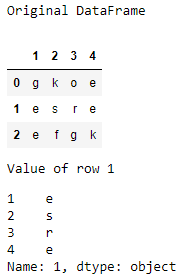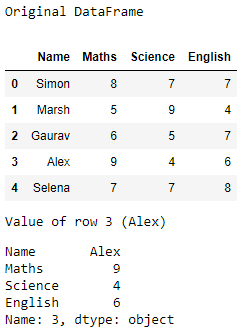# Get a specific row in a given Pandas DataFrame

In the Pandas DataFrame  we can find the specified row value with the using function iloc(). In this function we pass the row number as parameter.

## pandas.DataFrame.iloc[]

Syntax : pandas.DataFrame.iloc[]
Parameters :

• Index Position : Index position of rows in integer or list of integer.

Return type : Data frame or Series depending on parameters

Example 1 :

 `# importing the module ` `import` `pandas as pd ` ` `  `# creating a DataFrame ` `data ``=` `{``'1'` `: [``'g'``, ``'e'``, ``'e'``],  ` `        ``'2'` `: [``'k'``, ``'s'``, ``'f'``],  ` `        ``'3'` `: [``'o'``, ``'r'``, ``'g'``],  ` `        ``'4'` `: [``'e'``, ``'e'``, ``'k'``]} ` `df ``=` `pd.DataFrame(data) ` `print``(``"Original DataFrame"``) ` `display(df) ` ` `  `print``(``"Value of row 1"``) ` `display(df.iloc[``1``]) `

Output :Example 2:

 `# importing the module ` `import` `pandas as pd ` ` `  `# creating a DataFrame ` `data ``=` `{``'Name'` `: [``'Simon'``, ``'Marsh'``, ``'Gaurav'``,  ` `                 ``'Alex'``, ``'Selena'``],   ` `        ``'Maths'` `: [``8``, ``5``, ``6``, ``9``, ``7``],   ` `        ``'Science'` `: [``7``, ``9``, ``5``, ``4``, ``7``],  ` `        ``'English'` `: [``7``, ``4``, ``7``, ``6``, ``8``]}  ` `df ``=` `pd.DataFrame(data) ` `print``(``"Original DataFrame"``) ` `display(df) ` ` `  `print``(``"Value of row 3 (Alex)"``) ` `display(df.iloc[``3``]) `

Output :Attention geek! Strengthen your foundations with the Python Programming Foundation Course and learn the basics.

To begin with, your interview preparations Enhance your Data Structures concepts with the Python DS Course.

My Personal Notes arrow_drop_upCheck out this Author's contributed articles.

If you like GeeksforGeeks and would like to contribute, you can also write an article using contribute.geeksforgeeks.org or mail your article to contribute@geeksforgeeks.org. See your article appearing on the GeeksforGeeks main page and help other Geeks.

Please Improve this article if you find anything incorrect by clicking on the "Improve Article" button below.

Article Tags :

Be the First to upvote.

Please write to us at contribute@geeksforgeeks.org to report any issue with the above content.